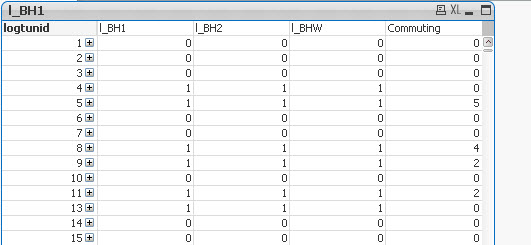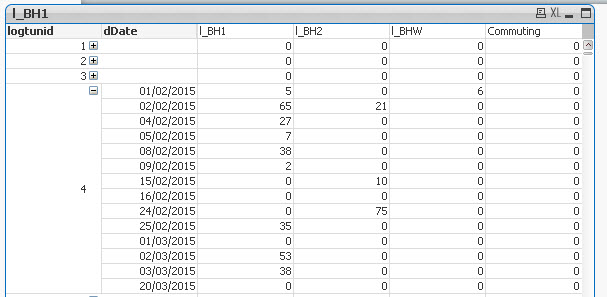# QlikView App Development

Discussion Board for collaboration related to QlikView App Development.

Announcements
Leverage your QlikView investment to modernize BI – see how! Join Group
cancel
Showing results for
Did you mean:
HighlightedPartner

## Pivot Table - Partial Sum is not working for Expression

Hello,

I am having problems in displaying sums for an expression in the Pivot Table.  The sum for other expressions (l_BH1, l_BH2, l_HW1) but for Commuting it is not displaying.

For l_BH1, l_BH2, l_HW1 the expressions are as follows:

=sum(if(nHour >= \$(vMin1) and nHour < \$(vMax1) and tWorkingDay = 1,1,0))

=sum(if(nHour >= \$(vMin2) and nHour < \$(vMax2) and tWorkingDay = 1,1,0))

=sum(if(nHour >= \$(vMin) and nHour < \$(vMax) and tWorkingDay = 1,1,0))

for Commuting the expression is as follows:

( I am using the expression Labels for l_BH1 and others in the commuting expression)

=if(((l_BH1) + (l_BH2))=0 and (l_HW1)>=1,1,0)

When I open the pivot for the dates I am able to see 1 and 0 for commuting but when i hide the pivot it does not show the sum for Commuting.  (in the image below it should show 3 for commuting).

Will appreciate if someone can assist me.

Thanks.Tags (4)
1 Solution

Accepted Solutions
HighlightedMVP & Luminary

## Re: Pivot Table - Partial Sum is not working for Expression

That's because =if(((l_BH1) + (l_BH2))=0 and (l_HW1)>=1,1,0) cannot be evaluated at the lotunid dimension level.

Try creating three variables:

vl_BH1: sum({<nHour={">=\$(vMin1)<\$(vMax1)"},tWorkingDay={1}>}1)

vl_BH2: sum({<nHour={">=\$(vMin2)<\$(vMax2)"},tWorkingDay={1}>}1)

vl_BHW: sum({<nHour={">=\$(vMin)<\$(vMax)"},tWorkingDay={1}>}1)

Use the variables for the expressions:

l_BH1: \$(vl_BH1)

l_BH2: \$(vl_BH2)

l_BHW: \$(vl_BHW)

And for the Commuting expression:

sum(aggr(if((\$(vl_BH1) + \$(vl_BH2))=0 and \$(vl_HW1)>=1,1,0),logtunid,dDate))

talk is cheap, supply exceeds demand
3 Replies
HighlightedMVP & Luminary

## Re: Pivot Table - Partial Sum is not working for Expression

That's because =if(((l_BH1) + (l_BH2))=0 and (l_HW1)>=1,1,0) cannot be evaluated at the lotunid dimension level.

Try creating three variables:

vl_BH1: sum({<nHour={">=\$(vMin1)<\$(vMax1)"},tWorkingDay={1}>}1)

vl_BH2: sum({<nHour={">=\$(vMin2)<\$(vMax2)"},tWorkingDay={1}>}1)

vl_BHW: sum({<nHour={">=\$(vMin)<\$(vMax)"},tWorkingDay={1}>}1)

Use the variables for the expressions:

l_BH1: \$(vl_BH1)

l_BH2: \$(vl_BH2)

l_BHW: \$(vl_BHW)

And for the Commuting expression:

sum(aggr(if((\$(vl_BH1) + \$(vl_BH2))=0 and \$(vl_HW1)>=1,1,0),logtunid,dDate))

talk is cheap, supply exceeds demand
HighlightedPartner

## Re: Pivot Table - Partial Sum is not working for Expression

Gysbert,

Thank you very much ... The commuting numbers are appearing perfectly.  Just one question I have.  The other expressions (l_BH1, l_BH2, l_HW1) are only being displayed when I click on the dDate pivot - otherwise it is showing 0/1.

Thank you very much.  Working perfectly!MVP & Luminary

## Re: Pivot Table - Partial Sum is not working for Expression

Perhaps these expressions will work better:

vl_BH1: count({<nHour={">=\$(vMin1)<\$(vMax1)"},tWorkingDay={1}>}logtunid)

vl_BH2: count({<nHour={">=\$(vMin2)<\$(vMax2)"},tWorkingDay={1}>}logtunid)

vl_BHW: count({<nHour={">=\$(vMin)<\$(vMax)"},tWorkingDay={1}>}logtunid)

talk is cheap, supply exceeds demand